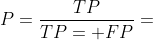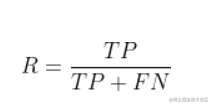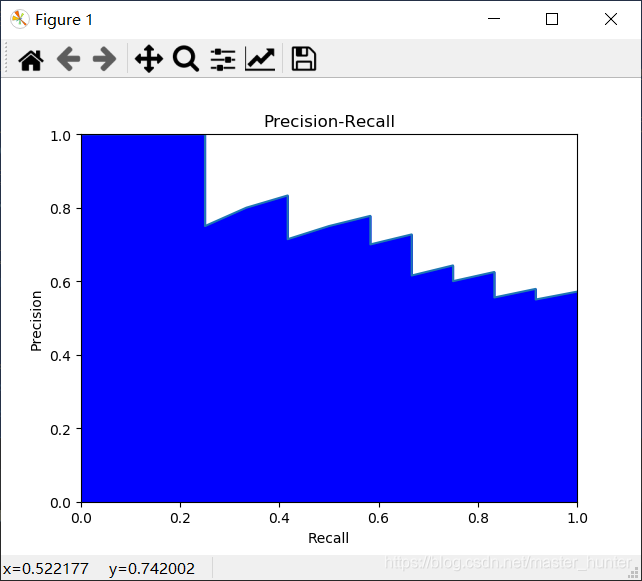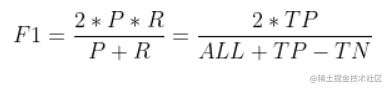# 一、性能度量

## 2.查准率、查全率与F1

      真实情况                                    预测结果
正例                   反例
正例             TP（真正例）               FN（假反例）
反例             FP（假正例）               TN（真反例）# 二、代码实现：

## 1.基于具体二分类问题算法实现代码：

 import numpy import matplotlib from matplotlib import pyplot as plt

# true = [真实组1，真实组2...真实组N]，predict = [预测组1，预测组2...预测组N]

def evaluation(true, predict): num = len(true) # 确定有几组 (TP, FP, FN, TN) = ( * num for i in range(4)) # 赋初值

for m in range(0, len(true)):
if (len(true[m]) != len(predict[m])):  # 样本数都不等，显然是有错误的
print("真实结果与预测结果样本数不一致。")
else:
for i in range(0, len(true[m])):  # 对每一组数据分别计数
if (predict[m][i] == 1) and ((true[m][i] == 1)):
TP[m] += 1.0
elif (predict[m][i] == 1) and ((true[m][i] == 0)):
FP[m] += 1.0
elif (predict[m][i] == 0) and ((true[m][i] == 1)):
FN[m] += 1.0
elif (predict[m][i] == 0) and ((true[m][i] == 0)):
TN[m] += 1.0
(P, R) = ( * num for i in range(2))
for m in range(0, num):
if (TP[m] + FP[m] == 0):
P[m] = 0  # 预防一些分母为0的情况
else:
P[m] = TP[m] / (TP[m] + FP[m])
if (TP[m] + FN[m] == 0):
R[m] = 0  # 预防一些分母为0的情况
else:
R[m] = TP[m] / (TP[m] + FN[m])

plt.title("P-R")
plt.xlabel("P")
plt.ylabel("R")
#plt.plot(P, R)
#plt.show()


if name == "main": # 简单举例

myarray_ture = numpy.random.randint(0, 2, (3, 100))
myarray_predict = numpy.random.randint(0, 2, (3, 100))
evaluation(myarray_ture,myarray_predict)




## 2.利用鸢尾花绘制P-R曲线

 from sklearn import svm, datasets from sklearn.model_selection import train_test_split import numpy as np iris = datasets.load_iris()

x = iris.data

y = iris.target

# target是一个数组，存储了data中每条记录属于哪一类鸢尾植物，所以数组的长度是150,所有不同值只有三个

random_state = np.random.RandomState(0)

# 给定状态为0的随机数组

n_samples, n_features = x.shape x = np.c_[x, random_state.randn(n_samples, 200 * n_features)]

# 添加合并生成特征测试数据集

x_train, x_test, y_train, y_test = train_test_split(x[y < 2], y[y < 2], test_size=0.25, random_state=0)

# 根据此模型训练简单数据分类器

classifier = svm.LinearSVC(random_state=0)#线性分类支持向量机 classifier.fit(x_train, y_train) y_score = classifier.decision_function(x_test)

from sklearn.metrics import precision_recall_curve import matplotlib.pyplot as plt

precision, recall, _ =precision_recall_curve(y_test, y_score) plt.fill_between(recall, precision,color='b') plt.xlabel('Recall') plt.ylabel('Precision') plt.ylim([0.0, 1.0]) plt.xlim([0.0, 1.0]) plt.plot(recall, precision) plt.title("Precision-Recall")

plt.show() 

### 效果：P-R图直观的显示出学习器在样本上的查全率、查准率。在进行比较时，若一个休息区的P-R曲线被另一个学习器的曲线完全“包住”，则可断言后者的性能优于前者。为取得比较合理的判断依据，将采用“平衡点”（Break-Even Point，BEP）度量对比算法的泛华性能强弱。它是“查准率=查全率”时的取值。但BEP还是过于简化，更常用F1度量（all为样例总数）：